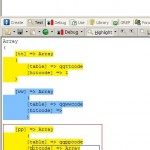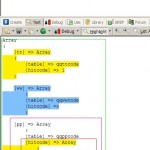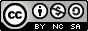# CFC4N小试正则表达式

Array
(
[tt] => Array
(
[table] => qqttcode
[hitcode] => 1
)

[ww] => Array
(
[table] => qqwwcode
[hitcode] =>
)

[pp] => Array
(
[table] => qqppcode
[hitcode] => Array
(
[table] => qqppcode
[hitcode] =>
)
)

)


CFC4N给出一下结果：

$strRge1 = '/($([^]]+)]\s?=>\s?)?Array[\s\S]+?$$([^()]|(?R))*$$/i'; arrReturn = array(); if (preg_match_all(strRge1,str,tt1)) { arrReturn = getarray(tt1); } arrReturn2 = array(); foreach (arrReturn as k => v) { arrReturn2[k] = v[k]; } print_r(arrReturn2); function getarray (strContents) { arrTemp = array(); strRge = '/\[([^]]+)]\s?=>\s?Array[\s\S]+?$$([^()]|(?R))*$$/i'; strReg2 = '/\[([^]]+?)]\s?=>\s?([\d\w]+)?/'; if (preg_match_all(strRge,strContents,strTemp)) { num = count(strTemp); if (num > '1') { for (i=0; i<num; i++) { if (preg_match_all(strRge,strTemp[i],arrTTT)) { arrTemp[strTemp[i]] = array(); arrTemp[strTemp[i]] = getarray(strTemp[i]); } else { arrTemp[strTemp[i]] = strTemp[i]; } } } else { arrTemp[strTemp] = array(); arrTemp2 = array(); if (preg_match_all(strReg2, strTemp,straa)) { num = count(straa); for (i=0; i<num-1; i++) { arrTemp2[straa[i+1]] = straa[i+1]; } } arrTemp[strTemp] = arrTemp2; } } return arrTemp; }  结果是可以用的。但是发现其只能用于固定的三层嵌套，假如N层的话，无法用这个函数了，后来，我又改造一下那个正则，改为 strRge1 = '/\[(([^]]+)]\s?=>\s?Array[\s]+?$$([^()])+|(?R))$$+/i';  但是，并不能解决问题。。各位看官，您认为，我的误区在哪里呢？ 附 第一个正则截图更改后正则匹配截图朋友乙：要求批量给html字符串中a标签中不包含title属性的标签添加title，而且，其title内容为<a href…>到</a>之间的文本。。 CFC4N给出答案为: str = '<a >ssss</a><a href="ss" >ssss</a><a title="ss" >ssss</a><a href="">ssss</a><a title="ss">ssss</a><a title="ss">ssss</a><a title="ssf">ssss</a>'; str = preg_replace('%<a((?:(?!title="[^"]+?")[\s\S])+?)>(?:(?<!</a>)[\s\S])+?</a>%im','<a title="\\2" \\1>\\2</a>',str); print_r(str);  各位看官，您认为，CFC4N写的正则表达式里，哪些还可以优化呢？这个效率是不是不高？？ 朋友丙:要求过滤非本域名，或者非本子域名的其他域名的UBB标签链接，一旦包含，直接替换成其中间的文本，比如例子字符串如下 [url=http://www.sadas.cn]baidu[/url] [url=www.ggasdwe.com]百度[/url] [url=http://www.qq.com/index.php]QQ[/url] [url=http://www.miyifun.com/index.html]其他 [/url] [url=pc.qq.com/index.php]PC QQ[/url]  其中，字符串中不确定有几个换行等其他字符，而且，不确定url的UBB标签中的网址中是否包含http://，不确定二级域名或者三级域名 CFC4N给出的正则以及PHP代码如下 str = '[url=http://www.sadas.cn]baidu[/url] [url=www.ggasdwe.com]百度[/url] [url=http://www.qq.com/index.php]QQ[/url] [url=http://www.miyifun.com/index.html]其他 [/url] [url=pc.qq.com/index.php]PC QQ[/url]'; print_r(preg_replace('%\[url=(http://)?(?:(?!qq\.com)[^$])*\][\r|\r\n]*([\s\S]+?)[\r|\r\n]*$/url$%i','\\2',$str));


squid的配置文件内容
CFC4N给出正则代码如下

preg_match_all('/^(?!#).+?$/m', file_get_contents('squid.conf'),$regs);
print_r(\$regs);


PS：以上正则，均为PCRE引擎。。其中，PHP代码的正则递归(迭代)部分，仅限于支持递归正则的引擎代码适用。。CFC4N的博客CFC4N 创作，采用 知识共享 署名-非商业性使用-相同方式共享（3.0未本地化版本）许可协议进行许可。基于https://www.cnxct.com上的作品创作。转载请注明转自：CFC4N小试正则表达式

### 3 thoughts on “CFC4N小试正则表达式”

1. 测试 表情 :shock:

2. 哈哈，多谢，多谢~